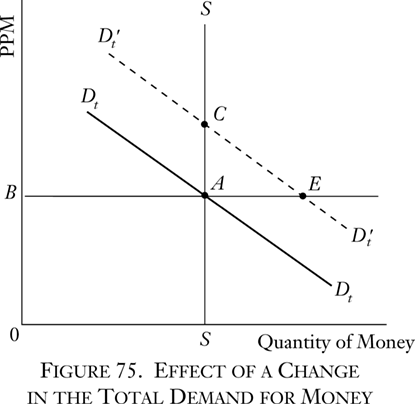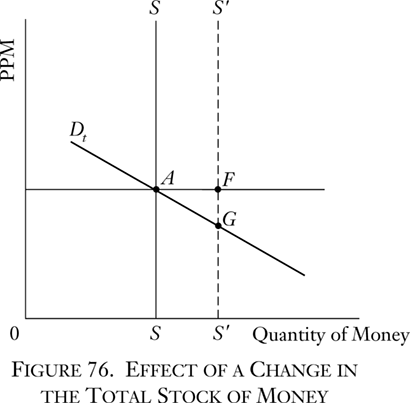# Books / Digital Text

## 3. Changes in the Money Relation

The purchasing power of money is therefore determined by two factors: the total demand schedule for money to hold and the stock of money in existence. It is easy to see on a diagram what happens when either of these determining elements changes. Thus, suppose that the schedule of total demand increases (shifts to the right). Then (see Figure 75) the total-demand-for-money curve has shifted from DtDt to DtDt′. At the previous equilibrium PPM point, A, the demand for money now exceeds the stock available by AE. The bids push the PPM upwards until it reaches the equilibrium point C. The converse will be true for a shift of the total demand curve leftward—a decline in the total demand schedule. Then, the PPM will fall accordingly.The effect of a change in the total stock, the demand curve remaining constant, is shown in Figure 76. Total quantity of stock increases from 0S to 0S′. At the new stock level there is an excess of stock, AF, over the total demand for money. Money will be sold at a lower PPM to induce people to hold it, and the PPM will fall until it reaches a new equilibrium point G. Conversely, if the stock of money is decreased, there will be an excess of demand for money at the existing PPM, and the PPM will rise until the new equilibrium point is reached.The effect of the quantity of money on its exchange-value is thus simply set forth in our analysis and diagrams.

The absurdity of classifying monetary theories into mutually exclusive divisions (such as “supply and demand theory,” “quantity theory,” “cash balance theory,” “commodity theory,” “income and expenditure theory”) should now be evident.4 For all these elements are found in this analysis. Money is a commodity; its supply or quantity is important in determining its exchange-value; demand for money for the cash balance is also important for this purpose; and the analysis can be applied to income and expenditure situations.

• 4. A typical such classification can be found in Lester V. Chandler, An Introduction to Monetary Theory (New York: Harper & Bros., 1940).